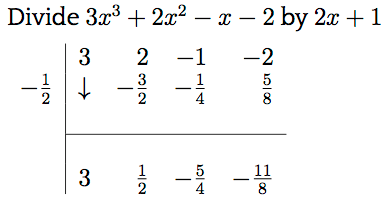Posted on

# A “Novel” approach to polynomial division…During the last few weeks I have been working with some Year 11 students from a local private school that has a fairly good reputation. These students have been working, in particular, on cubic and quartic functions, including the use of polynomial division. The students in question are taught by two different teachers at the school, including their school’s Head of Mathematics, a highly experienced and long serving teacher by all accounts. All of these students are certainly above average students, but like most of their peers, have had little exposure to the long division method in primary school, mostly due to the prevalence of handheld calculators.

Whilst working with these students, each of them has independently demonstrated to me a short-cut method taught to them at school for polynomial division. This short-cut method is well known and quite useful when applied correctly. Unfortunately, when originally demonstrated to the students (judging by each of their theory books), their respective teachers each made the same monumental error and taught the students a technique that would work only in certain circumstances. The following are some of the examples given to these students in class:

### Example 1From this example we can see that the top line contains the coefficients of the dividend (i.e.$x^{3} + x^{2} - 14x - 24$). The term on the extreme left of the second line ($-2$) is the value of$x$ that would make the divisor (i.e.$x + 2$) equal to zero. The remaining terms on the second line are this value multiplied by the results on the last line. The last line provides the coefficients of the quotient polynomial (i.e.$x^{2} - x - 12$) and remainder, which in this case is zero. Thus the solution is:$\left(x^{3} + x^{2} - 14x - 24\right) \div \left(x + 2\right) = x^{2} - x - 12$

In other words, the dividend expression can be factorised as:$\left(x^{3} + x^{2} - 14x - 24\right) = \left(x + 2\right) \left(x^{2} - x - 12\right)$

Of course the latter bracketed expression can be further factorised as$\left(x-4\right)\left(x+3\right)$  and so our final factorisation would be:$\left(x^{3} + x^{2} - 14x - 24\right) = \left(x + 2\right)\left(x-4\right)\left(x+3\right)$ .

So far, so good, but another example given to the students is where the major error was made. Here is another example, one that contains this major error:

### Example 2This example suggests that the quotient is$3x^{2} + \frac{1}{2}x - \frac{5}{4}$  with a remainder of$-\frac{11}{8}$ . Now most of you should hopefully be able to spot the problem with this. Clearly$3x^{3} \div 2x$  cannot possibly give a result of$3x^{2}$ , it must instead give a result of$\frac{3}{2}x^{2}$ . Further checking of the quotient will demonstrate that all of the coefficients are incorrect by a factor of two.

Naturally, as a concerned educator, I contacted the school in question to indicate that they were teaching (and worse assessing) a method that, in general, did not work and offered my assistance to the teachers involved in correcting the method. Unfortunately, the teachers involved, in particular the Head of Mathematics, did not even acknowledge my email and decided to instead defame me in their classes by suggesting that I did not know what I was doing. Furthermore, they blamed the students, suggesting that they had “missed a step” when copying down the example and proceeded to “re-demonstrate” the extra and supposedly missed step. If one student demonstrated this error I would potentially agree that this student had missed a step, but when multiple students demonstrate (independently) the same error, it can only be that they have learned it this way from their classroom teachers.

Of course, the “extra” step, is in fact an invented step designed to save face in front of the students. If one applies the known short-cut method correctly we would have:and thus the quotient would be$\frac{3}{2}x^{2} + \frac{1}{4}x - \frac{5}{8}$  with a remainder of$-\frac{11}{8}$ . Of course, in this short-cut, one multiplies the result in the last line by the numerator of the leftmost term, in this case minus one. After adding the next coefficient to this value, we divide the result by the denominator (i.e. two) to obtain the final value. So,$\frac{1}{4}$  is obtained by$\left[2 + \left(-1 \times \frac{3}{2}\right)\right] \div 2 = \frac{1}{4}$ .

It is a shame that these supposedly professional educators cannot acknowledge when they have made a mistake and demonstrate to their students the most valuable lesson of all, namely that we ALL make mistakes from time to time. What could have been an opportunity to teach students how to recognise mistakes was instead turned into a demonstration of backside covering and blame shifting. Mind you, I also found it insulting, laughable and at the same time quite sad that this Head of Mathematics would suggest that I don’t know what I am doing when (a) he does not know me, my background or experience at all; and (b) has no idea that it used to be part of my job to teach math to future math teachers and also to teach these future math teachers along with university academics HOW to teach. Oh well, I suppose it is teachers and academics like this and their mistakes and shortcomings that keep me in business.

First published 2013 | Last updated: 19 January 2018